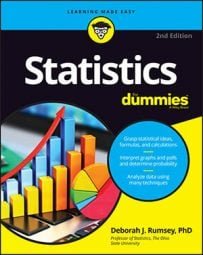##### Statistics For DummiesIn statistics, once you have calculated the slope and y-intercept to form the best-fitting regression line in a scatterplot, you can then interpret their values.

## Interpreting the slope of a regression line

The slope is interpreted in algebra as rise over run. If, for example, the slope is 2, you can write this as 2/1 and say that as you move along the line, as the value of the X variable increases by 1, the value of the Y variable increases by 2. In a regression context, the slope is the heart and soul of the equation because it tells you how much you can expect Y to change as X increases.

In general, the units for slope are the units of the Y variable per units of the X variable. It’s a ratio of change in Y per change in X. Suppose in studying the effect of dosage level in milligrams (mg) on systolic blood pressure (mmHg), a researcher finds that the slope of the regression line is –2.5. You can write this as –2.5/1 and say that systolic blood pressure is expected to decrease by 2.5 mmHg on average per 1 mg increase in drug dosage.

Always make sure to use proper units when interpreting slope. If you don’t consider units, you won’t really see the connection between the two variables at hand. For example, if Y is an exam score and X = study time, and you find the slope of the equation is 5, what does this mean? Not much without any units to draw from. Including the units, you see you get an increase of 5 points (change in Y) for every 1-hour increase in studying (change in X). Also be sure to watch for variables that have more than one common unit, such as temperature being in either Fahrenheit or Celsius; know which unit is being used.

If using a 1 in the denominator of slope is not super-meaningful to you, you can multiply the top and bottom by any number (as long as it’s the same number) and interpret it that way instead. In the systolic blood pressure example, instead of writing slope as –2.5/1 and interpreting it as a drop of 2.5 mmHg per 1 mg increase of the drug, you can multiply the top and bottom by 10 to get –25/10 and say an increase in dosage of 10 mg results in a decrease in systolic blood pressure of 25 mmHg.

## Interpreting the y-intercept of a regression line

The y-intercept is the place where the regression line y = mx + b crosses the y-axis (where x = 0), and is denoted by b. Sometimes the y-intercept can be interpreted in a meaningful way, and sometimes not. This uncertainty differs from slope, which is always interpretable. In fact, between the two concepts of slope and y-intercept, the slope is the star of the show, with the y-intercept serving as the less-famous but still noticeable sidekick.

At times the y-intercept makes no sense. For example, suppose you use rain to predict bushels per acre of corn. You know if the data set contains a point where rain is 0, the bushels per acre must be 0 as well. As a result, if the regression line crosses the y-axis somewhere else besides 0 (and there is no guarantee it will cross at 0 — it depends on the data), the y-intercept will make no sense. Similarly, in this context a negative value of y (corn production) cannot be interpreted.

Another situation where you can’t interpret the y-intercept is when data are not present near the point where x = 0. For example, suppose you want to use students’ scores on Midterm 1 to predict their scores on Midterm 2. The y-intercept represents a prediction for Midterm 2 when the score on Midterm 1 is 0. You don’t expect scores on a midterm to be at or near 0 unless someone didn’t take the exam, in which case her score wouldn’t be included in the first place.

Many times, however, the y-intercept is of interest to you, it has meaning, if you have data collected in the area where x = 0. For example, if you’re predicting coffee sales at football games in Green Bay, Wisconsin, using temperature, some games get cold enough to have temperatures at or even below 0 degrees Fahrenheit, so predicting coffee sales at these temperatures makes sense. (As you may guess, they sell more and more coffee as the temperature dips.)

When using a regression line, you can only apply the interpretations of the slope and y-intercept over the range of x values. It is dangerous to make predictions or statements beyond the scope of what you observed in the data set. Doing so is known as extrapolation. For example, suppose you collect data on the heights of children ages 2 to 8, and you calculate a slope of 3.7 inches per year. Thus, on average, these people grow 3.7 inches every year. But should we use that same value of slope to predict their height later in life as teenagers or even adults? Definitely not.